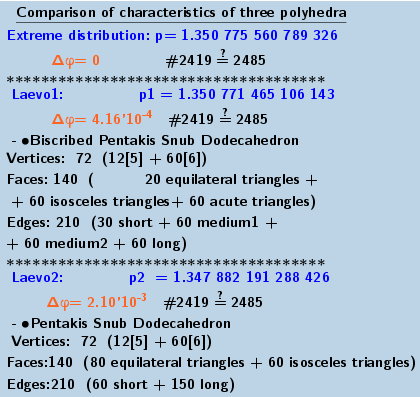# Coloring the edges and faces of a polyhedron(n=72) Pentakis Snub Dodecahedron (laevo) and its dual image.

Is considered as an example of the distribution of n=72 points on the surface of a sphere. In the applet, you can explore their extreme distribution. Two known distributions: Biscribed Pentakis Snub Dodecahedron (laevo), Pentakis Snub Dodecahedron (laevo). -are not extreme(in terms of the extreme value of the Distance Sum - sum of their mutual distances). Coloring of edges and faces of these polyhedra in applets: Extreme distribution Biscribed Pentakis Snub Dodecahedron (laevo) Pentakis Snub Dodecahedron (laevo)﻿ .Biscribed Pentakis Snub Dodecahedron (laevo)﻿ Pentakis Snub Dodecahedron (laevo)